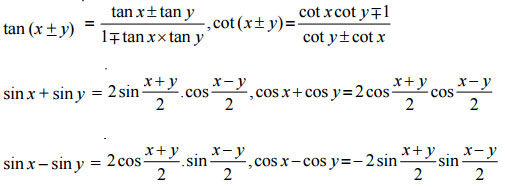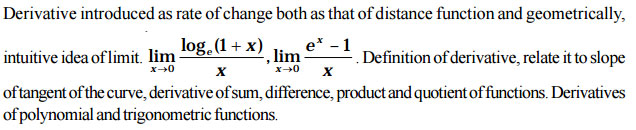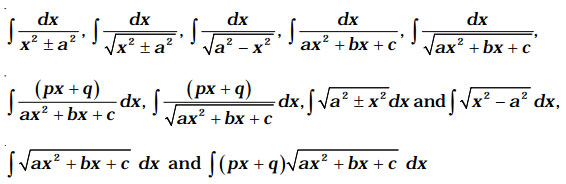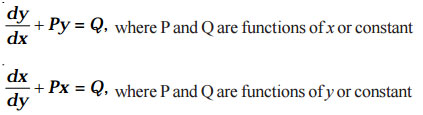# (Download) NCERT Revised Syllabus of Mathematics (Class 11 & 12)

Disclaimer: This website is NOT associated with CBSE, for official website of CBSE visit - www.cbse.gov.in

## MATHEMATICS (CLASSES XI –XII)

### General Guidelines

(i) All concepts/identities must be illustrated by situational examples.
(ii) The language of ‘word problems’ must be clear, simple and unambiguous.
(iii) Problems given should be testing the understanding of the subject.
(iv) All proofs to be produced in a manner that allow the learner to see flow of reasons. Wherever possible, give more than one proof.
(v) Motivate results, wherever possible. Prove explicitly those results where a short and clear argument reinforces mathematical thinking and reasoning. There must be emphasis on correct way of expressing the arguments.

## CLASS XI

### (Periods 12)

Sets and their representations. Empty set. Finite and Infinite sets. Equal sets. Subsets. Subsets of the set of real numbers especially intervals (with notations). Power set. Universal set. Venn diagrams. Union and intersection of sets. Difference of sets. Complement of a set, Properties of Complement sets.

### (Periods 14)

Ordered pairs, Cartesian product of sets. Number of elements in the Cartesian product of two finite sets. Cartesian product of the reals with itself (upto R × R × R).
Definition of relation, pictorial diagrams, domain, co-domain and range of a relation. Function as a special kind of relation from one set to another. Pictorial representation of a function, domain, co-domain and range of a function. Real valued function of the real variable, domain and range of these functions, constant, identity, polynomial, rational, modulus, signum and greatest integer functions with their graphs. Sum, difference, product and quotients of functions.

### (Periods 18)

Positive and negative angles. Measuring angles in radians and in degrees and conversion from one measure to another. Definition of trigonometric functions with the help of unit circle. Truth of the identity sin2 x + cos2 x = 1, for all x. Signs of trigonometric functions and sketch of their graphs. Expressing sin (x+ y) and cos (x + y) in terms of sin x, sin y, cos x and cos y. Deducing the identities like following:Identities related to sin2x, cos2x, tan2x, sin3x, cos3x and tan3x. General solution of trigonometric equations of the type sinθ = sinα, cosθ = cosα and tanθ = tanα. Proofs and simple applications of sine and cosine formulae.

## UNIT II : ALGEBRA

### (Periods 06)

Process of the proof by induction, motivating the application of the method by looking at natural numbers as the least inductive subset of real numbers. The principle of mathematical induction and simple applications.

### (Periods 10)

Linear inequalities, Algebraic solutions of linear inequalities in one variable and their representation on the number line. Graphical solution of linear inequalities in two variables. Solution of system of linear inequalities in two variables - graphially.

### (Periods 12)

Fundamental principle of counting. Factorial n. Permutations and combinations derivation of formulae and their connections, simple applications.

### (Periods 08)

History, statement and proof of the binomial theorem for positive integral indices. Pascal’s triangle, general and middle term in binomial expansion, simple applications.

### (Periods 10)

Sequence and Series. Arithmetic Progression (A.P.), Arithmetic Mean (A.M.), Geometric Progression (G.P.), general term of a G.P., sum of n terms of a G.P. Arithmetic and geometric series, infinite G.P. and its sum, geometric mean (G.M.). Relation between A.M. and G.M. Sum
to n terms of the special series : ∑ n, ∑ n2 and ∑ n3

## UNIT III : COORDINATE GEOMETRY

### (Periods 09)

Brief recall of 2-D from earlier classes, shifting of origin. Slope of a line and angle between two lines. Various forms of equations of a line: parallel to axes, point-slope form, slope-intercept form, two-point form, intercepts form and normal form. General equation of a line. Equation of family of lines passing through the point of intersection of two lines. Distance of a point from a line.

### (Periods 12)

Sections of a cone: Circles, ellipse, parabola, hyperbola, a point, a straight line and pair of intersecting lines as a degenerated case of a conic section. Standard equations and simple properties of parabola, ellipse and hyperbola. Standard equation of a circle.

### (Periods 08)

Coordinate axes and coordinate planes in three dimensions. Coordinates of a point. Distance between two points and section formula.

## UNIT IV : CALCULUS

### (Periods 18)## UNIT V: MATHEMATICAL REASONING

### (Periods 08)

Mathematically acceptable statements. Connecting words/phrases - consolidating the understanding of “if and only if (necessary and sufficient) condition”, “implies”, “and/or”, “implied by”, “and”, “or”, “there exists” and their use through variety of examples related to real life and Mathematics. Validating the statements involving the connecting words - difference between contradiction, converse and contrapositive.

## UNIT VI : STATISTICS AND PROBABILITY

### (Periods 10)

Measure of dispersion; mean deviation, variance and standard deviation of ungrouped/grouped data. Analysis of frequency distributions with equal means but different variances.

### (Periods 15)

Random experiments: outcomes, sample spaces (set representation). Events: Occurrence of events, ‘not’, ‘and’ & ‘or’ events, exhaustive events, mutually exclusive events. Axiomatic (set theoretic) probability, connections with the theories of earlier classes. Probability of an event, probability of ‘not’, ‘and’, & ‘or’ events.

## UNIT I: RELATIONS AND FUNCTIONS

### (Periods 10)

Types of relations: Reflexive, symmetric, transitive and equivalence relations. One to one and onto functions, composite functions, inverse of a function. Binary operations.

### (Periods 12)

Definition, range, domain, principal value branches. Graphs of inverse trigonometric functions.
Elementary properties of inverse trigonometric functions.

## UNIT II: ALGEBRA

### (Periods 18)

Concept, notation, order, equality, types of matrices, zero matrix, transpose of a matrix, symmetric and skew symmetric matrices. Addition, multiplication and scalar multiplication of matrices, simple properties of addition, multiplication and scalar multiplication. Non-commutativity of multiplication of matrices and existence of non-zero matrices whose product is the zero matrix (restrict to square matrices of order 2). Concept of elementary row and column operations. Invertible matrices and
proof of the uniqueness of inverse, if it exists; (Here all matrices will have real entries).

### (Periods 20)

Determinant of a square matrix (up to 3 × 3 matrices), properties of determinants, minors, cofactors and applications of determinants in finding the area of a triangle. Adjoint and inverse of a square matrix. Consistency, inconsistency and number of solutions of system of linear equations by examples, solving system of linear equations in two or three variables (having unique solution) using inverse of a matrix.

## UNIT III: CALCULUS

### (Periods 18)

Continuity and differentiability, derivative of composite functions, chain rule, derivatives of inverse trigonometric functions, derivative of implicit function. Concepts of exponential, logarithmic functions. Derivatives of loge
x
and ex
. Logarithmic differentiation. Derivative of functions expressed in parametric
forms. Second order derivatives. Rolle’s and Lagrange’s Mean Value Theorems (without proof)
and their geometric interpretations.

### (Periods 10)

Applications of derivatives: Rate of change, increasing/decreasing functions, tangents and normals, approximation, maxima and minima (first derivative test motivated geometrically and second derivative test given as a provable tool). Simple problems (that illustrate basic principles and understanding of the subject as well as real-life situations).

### (Periods 20)

Integration as inverse process of differentiation. Integration of a variety of functions by substitution,
by partial fractions and by parts, only simple integrals of the type –to be evaluated.
Definite integrals as a limit of a sum. Fundamental Theorem of Calculus (without proof). Basic
properties of definite integrals and evaluation of definite integrals.

### (Periods 10)

Applications in finding the area under simple curves, especially lines, arcs of circles/parabolas/ellipses (in standard form only), area between the two above said curves (the region should be cleraly identifiable).

### (Periods 10)

Definition, order and degree, general and particular solutions of a differential equation. Formation of differential equation whose general solution is given. Solution of differential equations by method of separation of variables, homogeneous differential equations of first order and first degree. Solutions of linear differential equation of the type –## UNIT IV: VECTORS AND THREE-DIMENSIONAL GEOMETRY

### (Periods 10)

Vectors and scalars, magnitude and direction of a vector. Direction cosines/ratios of vectors. Types of vectors (equal, unit, zero, parallel and collinear vectors), position vector of a point, negative of a vector, components of a vector, addition of vectors, multiplication of a vector by a scalar, position vector of a point dividing a line segment in a given ratio. Scalar (dot) product of vectors, projection of a vector on a line. Vector (cross) product of vectors, scalar triple product.

### (Periods 12)

Direction cosines/ratios of a line joining two points. Cartesian and vector equation of a line, coplanar and skew lines, shortest distance between two lines. Cartesian and vector equation of a plane. Angle between (i) two lines, (ii) two planes, (iii) a line and a plane. Distance of a point from a plane.

## Unit V: Linear Programming

### (Periods 12)

Introduction, related terminology such as constraints, objective function, optimization, different types of linear programming (L.P.) problems, mathematical formulation of L.P. problems, graphical method of solution for problems in two variables, feasible and infeasible regions, feasible and infeasible solutions, optimal feasible solutions (up to three non-trivial constrains).

## Unit VI: Probability

### (Periods 18)

Multiplications theorem on probability. Conditional probability, independent events, total probability, Baye’s theorem. Random variable and its probability distribution, mean and variance of haphazard variable. Repeated independent (Bernoulli) trials and Binomial distribution.